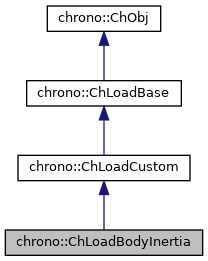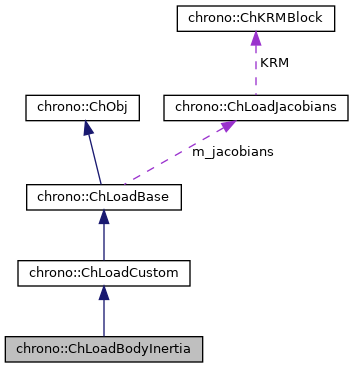## Description

Note that a ChBody already has a mass an inertia tensor, however for speed optimization reasons the ChBody mass is limited to zero offset of center of mass, moreover it does not generate a gyroscopical damping matrix. Therefore, you can use this load if you need:

• an offset between the body and its center of mass
• a gyroscopic damping matrix (and inertial stiffness matrix), ex. it may affect for advanced aeroelastic modal analysis. Note that you must use the solvers for finite elements in order to exploit this feature.

#include <ChLoadsBody.h>[legend][legend]

## Public Member Functions

ChLoadBodyInertia (std::shared_ptr< ChBody > body, const ChVector<> &m_offset, const double m_mass, const ChVector<> &m_IXX=VNULL, const ChVector<> &m_IXY=VNULL)

"Virtual" copy constructor (covariant return type).

void SetInertia (const ChMatrix33<> &newXInertia)
Set the inertia tensor of the body, assumed in the body reference basis, with body reference as center. More...

const ChMatrix33GetInertia () const
Set the inertia tensor of the body, assumed in the body reference basis, with body reference as center. More...

void SetInertiaXX (const ChVector<> &iner)
Set the diagonal part of the inertia tensor (Ixx, Iyy, Izz values). More...

ChVector GetInertiaXX () const
Get the diagonal part of the inertia tensor (Ixx, Iyy, Izz values). More...

void SetInertiaXY (const ChVector<> &iner)
Set the off-diagonal part of the inertia tensor (Ixy, Ixz, Iyz values). More...

ChVector GetInertiaXY () const
Get the extra-diagonal part of the inertia tensor (Ixy, Ixz, Iyz values). More...

virtual void ComputeQ (ChState *state_x, ChStateDelta *state_w) override
Compute Q, the generalized load. More...

virtual void ComputeJacobian (ChState *state_x, ChStateDelta *state_w, ChMatrixRef mK, ChMatrixRef mR, ChMatrixRef mM) override
For efficiency reasons, do not let the parent class do automatic differentiation to compute the R, K matrices. More...

virtual void LoadIntLoadResidual_Mv (ChVectorDynamic<> &R, const ChVectorDynamic<> &w, const double c) override
Just for efficiency, override the default LoadIntLoadResidual_Mv, because we can do this in a simplified way. More...Public Member Functions inherited from chrono::ChLoadCustom

Gets the number of DOFs affected by this load (position part)

Gets the number of DOFs affected by this load (speed part)

virtual void LoadGetStateBlock_x (ChState &mD) override
Gets all the current DOFs packed in a single vector (position part)

virtual void LoadGetStateBlock_w (ChStateDelta &mD) override
Gets all the current DOFs packed in a single vector (speed part)

virtual void LoadStateIncrement (const ChState &x, const ChStateDelta &dw, ChState &x_new) override
Increment a packed state (ex. More...

Number of coordinates in the interpolated field, ex=3 for a tetrahedron finite element or a cable, = 1 for a thermal problem, etc.

Adds the internal loads Q (pasted at global nodes offsets) into a global vector R, multiplied by a scaling factor c, as R += forces * c.

virtual void CreateJacobianMatrices () override
Create the jacobian loads if needed, and also set the ChVariables referenced by the sparse KRM block.

virtual ChVectorDynamicGetQ ()
Access the generalized load vector Q.Public Member Functions inherited from chrono::ChLoadBase
Access the jacobians (if any, i.e. if this is a stiff load)

virtual void Update (double time)
Update: this is called at least at each time step. More...

virtual void InjectKRMmatrices (ChSystemDescriptor &mdescriptor)
Tell to a system descriptor that there are item(s) of type ChKblock in this object (for further passing it to a solver)

virtual void KRMmatricesLoad (double Kfactor, double Rfactor, double Mfactor)
Adds the current stiffness K and damping R and mass M matrices in encapsulated ChKblock item(s), if any. More...Public Member Functions inherited from chrono::ChObj
ChObj (const ChObj &other)

int GetIdentifier () const
Gets the numerical identifier of the object.

void SetIdentifier (int id)
Sets the numerical identifier of the object.

double GetChTime () const
Gets the simulation time of this object.

void SetChTime (double m_time)
Sets the simulation time of this object.

const char * GetName () const
Gets the name of the object as C Ascii null-terminated string -for reading only!

void SetName (const char myname[])
Sets the name of this object, as ascii string.

std::string GetNameString () const
Gets the name of the object as C Ascii null-terminated string.

void SetNameString (const std::string &myname)
Sets the name of this object, as std::string.

void MFlagsSetAllOFF (int &mflag)

void MFlagsSetAllON (int &mflag)

void MFlagSetON (int &mflag, int mask)

void MFlagSetOFF (int &mflag, int mask)

int MFlagGet (int &mflag, int mask)

virtual void ArchiveOUT (ChArchiveOut &marchive)
Method to allow serialization of transient data to archives.

virtual void ArchiveIN (ChArchiveIn &marchive)
Method to allow de-serialization of transient data from archives.

virtual std::string & ArchiveContainerName ()Public Attributes inherited from chrono::ChLoadCustomProtected Attributes inherited from chrono::ChLoadBaseProtected Attributes inherited from chrono::ChObj
double ChTime
the time of simulation for the object

## Constructor & Destructor Documentation

 chrono::ChLoadBodyInertia::ChLoadBodyInertia ( std::shared_ptr< ChBody > body, const ChVector<> & m_offset, const double m_mass, const ChVector<> & m_IXX = VNULL, const ChVector<> & m_IXY = VNULL )
Parameters
 body object to apply additional inertia to m_offset offset of the center of mass, in body coordinate system m_mass added mass [kg] m_IXX added diag. inertia values Ixx, Iyy, Izz (in body coordinate system, centered in body) m_IXY added off.diag. inertia values Ixy, Ixz, Iyz including the "-"sign (in body coordinate system, centered in body)

## ◆ ComputeJacobian()

 void chrono::ChLoadBodyInertia::ComputeJacobian ( ChState * state_x, ChStateDelta * state_w, ChMatrixRef mK, ChMatrixRef mR, ChMatrixRef mM )
overridevirtual

For efficiency reasons, do not let the parent class do automatic differentiation to compute the R, K matrices.

Use analytic expressions instead. For example, R is the well known gyroscopic damping matrix. Also, compute the M matrix.

Parameters
 state_x state position to evaluate jacobians state_w state speed to evaluate jacobians mK result -dQ/dx mR result -dQ/dv mM result -dQ/da

## ◆ ComputeQ()

 void chrono::ChLoadBodyInertia::ComputeQ ( ChState * state_x, ChStateDelta * state_w )
overridevirtual

In this case, it computes the quadratic (centrifugal, gyroscopic) terms. Signs are negative as Q assumed at right hand side, so Q= -Fgyro -Fcentrifugal Called automatically at each Update(). The M*a term is not added: to this end one could use LoadIntLoadResidual_Mv afterward.

Parameters
 state_x state position to evaluate Q state_w state speed to evaluate Q

## ◆ GetInertia()

 const ChMatrix33& chrono::ChLoadBodyInertia::GetInertia ( ) const
inline

Set the inertia tensor of the body, assumed in the body reference basis, with body reference as center.

The return 3x3 symmetric matrix contains the following values:

 [ int{y^2+z^2}dm    -int{xy}dm    -int{xz}dm    ]
[                  int{x^2+z^2}   -int{yz}dm    ]
[       (symm.)                  int{x^2+y^2}dm ]


## ◆ GetInertiaXX()

Get the diagonal part of the inertia tensor (Ixx, Iyy, Izz values).

The vector contains these values, assumed in the body reference basis, with body reference as center:

[  int{y^2+z^2}dm   int{x^2+z^2}   int{x^2+y^2}dm ]


## ◆ GetInertiaXY()

Get the extra-diagonal part of the inertia tensor (Ixy, Ixz, Iyz values).

The vector contains these values, assumed in the body reference basis, with body reference as center:

[ -int{xy}dm   -int{xz}dm   -int{yz}dm ]


overridevirtual

Just for efficiency, override the default LoadIntLoadResidual_Mv, because we can do this in a simplified way.

a scaling factor

Parameters
 R result: the R residual, R += c*M*w w the w vector

## ◆ SetInertia()

 void chrono::ChLoadBodyInertia::SetInertia ( const ChMatrix33<> & newXInertia )

Set the inertia tensor of the body, assumed in the body reference basis, with body reference as center.

The provided 3x3 matrix should be symmetric and contain the inertia tensor as: system:

              [ int{y^2+z^2}dm    -int{xy}dm    -int{xz}dm    ]
newXInertia = [                  int{x^2+z^2}   -int{yz}dm    ]
[     (symm.)                    int{x^2+y^2}dm ]


## ◆ SetInertiaXX()

 void chrono::ChLoadBodyInertia::SetInertiaXX ( const ChVector<> & iner )

Set the diagonal part of the inertia tensor (Ixx, Iyy, Izz values).

The vector should contain these moments of inertia, assumed in the body reference basis, with body reference as center:

iner = [  int{y^2+z^2}dm   int{x^2+z^2}   int{x^2+y^2}dm ]


## ◆ SetInertiaXY()

 void chrono::ChLoadBodyInertia::SetInertiaXY ( const ChVector<> & iner )

Set the off-diagonal part of the inertia tensor (Ixy, Ixz, Iyz values).

The vector contains these values, assumed in the body reference basis, with body reference as center:

iner = [ -int{xy}dm   -int{xz}dm   -int{yz}dm ]


The documentation for this class was generated from the following files: